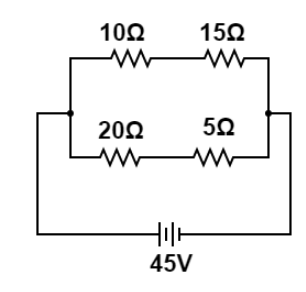# In the circuit shown below, calculate the net resistance of the circuit.Verified
124.2k+ views
Hint: In electrical circuits, resistance is the property of some materials which opposes the flow of current across them in the circuit and these types of materials are known as resistors. We will use the general formula of calculating net resistances when connected in series and when connected in parallel to each other.

Formula used:
When two resistors of resistance say ${R_1}(and){R_2}$ connected in series, then their net resistance can be calculated as ${R_{series}} = {R_1} + {R_2}$ whereas when these two same resistors are connected in parallel combination then, we can calculate their net resistance as $\dfrac{1}{{{R_{parallel}}}} = \dfrac{1}{{{R_1}}} + \dfrac{1}{{{R_2}}}$.

Let us first find the net resistance among the series combination of resistances $10\Omega (and)15\Omega$ let’s say net resistance among this series combination is ${R_{10,15}}$ now, using series formula ${R_{series}} = {R_1} + {R_2}$ we have,
${R_{10,15}} = 10 + 15$
$\Rightarrow {R_{10,15}} = 25\Omega \to (i)$
Now, again we have resistances of $20\Omega (and)5\Omega$ are connected in series so using again series formula ${R_{series}} = {R_1} + {R_2}$ , their net resistance can be written as,
${R_{20,5}} = 20 + 5$
$\Rightarrow {R_{20,5}} = 25\Omega \to (ii)$

Now, the net resistances of ${R_{10,15}}(and){R_{20,5}}$ both are connected in parallel combination, so using parallel combination formula $\dfrac{1}{{{R_{parallel}}}} = \dfrac{1}{{{R_1}}} + \dfrac{1}{{{R_2}}}$ we can find net resistance of circuits as:
$\dfrac{1}{{{R_{net}}}} = \dfrac{1}{{{R_{10,15}}}} + \dfrac{1}{{{R_{20,5}}}}$
Put the values from the equations ${R_{10,15}} = 25\Omega \to (i)$ and ${R_{20,5}} = 25\Omega \to (ii)$ we have,
$\dfrac{1}{{{R_{net}}}} = \dfrac{1}{{25}} + \dfrac{1}{{25}}$
$\Rightarrow \dfrac{1}{{{R_{net}}}} = \dfrac{2}{{25}}$
$\therefore {R_{net}} = 12.5\Omega$

Hence, the net resistance of the given circuit diagram is ${R_{net}} = 12.5\Omega$.

Note: It should be remembered that, when two resistances are connected in series combination, the current flowing across both resistances are same but potential difference is different. while in parallel combination, the potential difference across both resistances is same but different current flows across both the resistances.